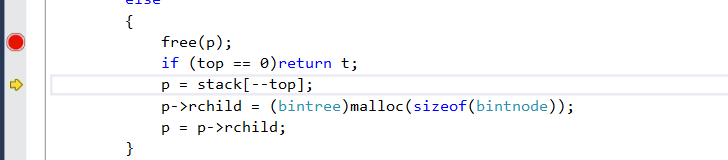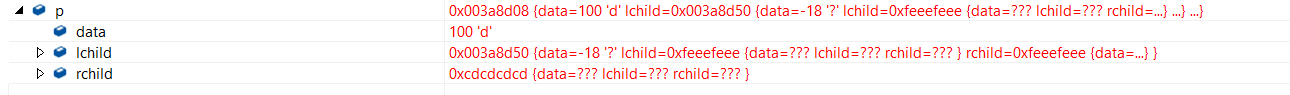'#'代表NULL,

``````bintree createbintree(char *s) {
bintree stack[Maxsize];
int top = 0;
bintree t = (bintree)malloc(sizeof(bintnode));
bintree p = t;
while ((*s)!='\0')
{
if ((*s)!='#')
{
p->data = *s;
stack[top++] = p;
p->lchild = (bintree)malloc(sizeof(bintnode));
p = p->lchild;
}
else
{
if(p!=NULL)
free(p);
if (top == 0)return t;
p = stack[--top];
p->rchild = (bintree)malloc(sizeof(bintnode));
p = p->rchild;
}
s++;
}
return t;
}
``````1个回答

``````#include "stdlib.h"

#define Maxsize 100

typedef struct bintnode
{
char data;
bintnode * lchild;
bintnode * rchild;
} *bintree;

bintree createbintree(char *s) {
bintree stack[Maxsize];
int top = 0;
bintree t = (bintree)malloc(sizeof(bintnode));
bintree p = t;
while ((*s) != '\0')
{
if ((*s) != '#')
{
p->data = *s;
stack[top++] = p;
p->lchild = (bintree)malloc(sizeof(bintnode));
p = p->lchild;
}
else
{
if (p != NULL)
{
//free(p);
}
if (top == 0)return t;
p = stack[--top];
p->rchild = (bintree)malloc(sizeof(bintnode));
p = p->rchild;
}
s++;
}
return t;
}

int main()
{
char * s = "abd#e##fg###c##";
bintree tree = createbintree(s);
return 0;
}
``````